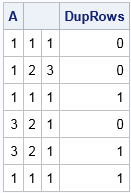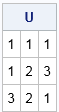Sometimes it is important to ensure that a matrix has unique rows. When the data are all numeric, there is an easy way to detect (and delete!) duplicate rows in a matrix.

The main idea is to subtract one row from another. Start with the first row and subtract it from every row beneath it. If any row of the difference matrix is identically zero, then you have found a row that is identical to the first row. Then do the same thing for the second row: subtract it from the third and higher rows and see if you obtain a row of zeros. Repeat this process for the third and higher rows.

The following SAS/IML program implements this algorithm for an arbitrary numeric matrix. It returns a binary indicator variable (a column vector). The i_th row of the returned vector is 1 for rows that are duplicates of some previous row:

```proc iml; /* return binary vector that indicates which rows in a numerical matrix are duplicates */ start DupRows(A); N = nrow(A); dupRows = j(N, 1, 0); /* binary indicator matrix */ do i = 1 to N-1; if dupRows[i] = 0 then do; /* skip rows that are known to be duplicates */ r = i+1:N; /* remaining rows */ M = A[r, ]-A[i, ]; /* subtract current row from remaining rows */ b = M[ ,##]; /* sum of squares = 0 iff duplicate row */ idx = loc( b=0 ); /* any duplicate rows for current row? */ if ncol(idx) > 0 then dupRows[r[idx]] = 1; /* if so, flag them */ end; end; return dupRows; finish;```

To test the function, consider the following 6 x 3 matrix. You can see by inspection that the matrix has three duplicate rows: the third, fifth, and sixth rows. You can call the DupRows function and print the matrix adjacent to the binary vector that indicates the duplicate rows:

```A = {1 1 1, 1 2 3, 1 1 1, /* duplicate row */ 3 2 1, 3 2 1, /* duplicate row */ 1 1 1}; /* duplicate row */ DupRows = DupRows(A); print A DupRows;```You can use the DupRows function to write a function that excludes the duplicate rows in a matrix and returns only the unique rows, as follows:

```/* return the unique rows in a numerical matrix */ start UniqueRows(A); uniqRows = loc(DupRows(A)=0); return(A[uniqRows, ]); /* return rows that are NOT duplicates */ finish;   U = UniqueRows(A); print U;```I like this algorithm because it uses subtraction, which is a very fast operation. However, This algorithm has a complexity of O(n(n-1)/2), where n is the number of rows in the matrix, so it is best for small to moderate values of n.

For long and skinny matrices (or for character data), it might be more efficient to sort the matrix as I showed in a previous article about how to find (and count) the unique rows of a matrix. However, the sorting algorithm requires that you sort the data by ALL columns, which can be inefficient for very wide and short data tables. For small and medium-sized data, you can use either method to find the unique rows in a matrix.

This article discusses how to remove duplicates records for numerical matrices in SAS/IML. In Base SAS, you can use PROC SORT or PROC SQL to remove duplicate records from a SAS DATA set, as shown in Lafler (2017) and other references.

The post Find the unique rows of a numeric matrix appeared first on The DO Loop.